## Notations

The Quantum Algebra extension uses a number of notations to improve the readability of expressions. They supplement the notations that already exist in the basic package. This also takes into account the need for documentation.

Notations of the basic package:

• Operator Notation
• Superdagger
• Centerdot

• Integral
• Total Notation
• Partial Derivative
• Small Circle

### Overview

 Name Sample Notation FullForm - Simplified or Complete Expression Operator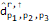`Operator[d, "H", `` List[Subscript[p, 1], Subscript[p, 2], Subscript[p, 3]], List]` SuperDagger`Hermitian[Operator[d, `` List[Subscript[p, 1], Subscript[p, 2], Subscript[p, 3]], List]]` CenterDot`NonCommutativeMultiply[Operator[d,"H",List[Subscript[p, 1]], List],``Operator[d, List[Subscript[p, 1]], List]]` QAIntegrate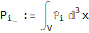`QAIntegrate[Subscript[p, i], V, 3, x]` QASum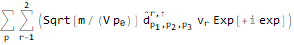`QASum[Operator[d, "H",  List[Subscript[p, 1], Subscript[p, 2], Subscript[p, 3]], List],p]` QAD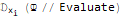`QAD[\[psi] // Evaluate, Subscript[x, i]]` SmallCircle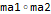` SmallCircle[ma1, ma2]`